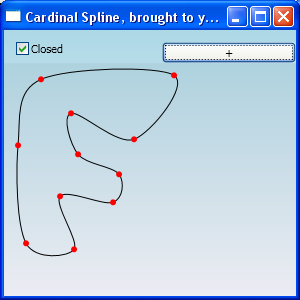## DrawCurve / AddCurve for WPF / Cardinal Spline

For my project I need to draw Cardinal splines. Preferable the same way I was used to with the System.Drawing.Graphics or System.Drawing.Drawing2D.GraphicsPath. Not knowing much about splines, I noticed the number of PathPoints on a graphics path. The curve is drawn with beziers, so it’s some sort of string of beziers that make up this cardinal spline.

This project results in the same curves as you are used to with the graphics object (but this works with doubles not with floats). Tension and open and closed params are supported!

Anyway long story short, this project calculates control points for beziers. The curve will be draw with beziers, thus the outcome is exactly the same as with the Graphics object. I’ve seen other examples drawing the curve by them self calculating 100’s of points on the bezier it self .

Yes the graphics object does the same thing see msdn (quote:”Remarks The user must keep the original points if they are needed. The original points are converted to cubic Bézier control points internally, therefore there is no mechanism for returning the original points.”) .

If you draw a curve with 3 points, 7 points are needed to draw the curve, thus a performance gain(? not measured!) without losing the nice smoothness of the curve.

here is a screen shot:comments are welcome, Can u use this, or are you missing something?

## Simple Calculator with Antlr.

For quite some time I’ve been interested in creating scripting languages to make existing applications more flexible than thought possible. After writing a couple of parsers I’ve stumbled upon ANTLR (ANother Tool for Language Recognition) which is a Lexer and Parser all in one.

Although I’m aware of the existence of a couple of other tools, such as “yacc, lex,gold parser,.. ” this is the first tool I’m going to try out. I see a appliance to read svg files, and generate code (c#/Java/vb/ada) that will draw the figure.

Here is a preview of what this sample aims to:

But first things first, I longed to create a simple calculator, helping me to asses the possibilities of ANTLR, with a little help from :The Definitive ANTLR Reference: Building Domain-Specific Languages (Pragmatic Programmers)(Rather helpfull), I’ve managed the job quite well.

Since I’ve seen some questions on the Internet requesting a simple example for a simple calculator grammar, here it is:

```grammar SimpleCalc;

tokens {
PLUS 	= '+' ;
MINUS	= '-' ;
MULT	= '*' ;
DIV	= '/' ;
RPAREN	= ')' ;
LPAREN	= '(' ;
ASSIGN	= '=' ;
}

/*----------------
* PARSER RULES
*----------------*/

prog :		stat+;
stat :		expr NEWLINE
|	ID ASSIGN expr NEWLINE
|	NEWLINE; 			//Do nothing

expr 	:	multExpr ((PLUS | MINUS )multExpr)*;

multExpr:	atom ((MULT | DIV) atom )*;

atom 	:	INT
|	ID
|	LPAREN expr RPAREN;

/*----------------
* LEXER RULES
*----------------*/

ID 	:	('a'..'z'|'A'..'Z')+;
INT 	:	'0'..'9'+;
NEWLINE :	'\r'?'\n';
WS 	:	(' '|'\t'|'\n'|'\r')+;```

The grammar above can handle expressions such as:

1+2*3
a=4-5
c=a*(6/2)

Now one has to add actions to the rules these will look like:

```atom returns[int value] <==Tell Antlr this rule is implemented as a function, returning an int. : INT {\$value = int.Parse(\$INT.text);} <==The return value '\$value' is assign the parsed as an integer string value of int. ```

Now all you need to do is fill every thing in. When an ID follow by an assignment (a=6) is encounter it will be placed in a Dictionary.

After adding the appropriate actions the calculator is finished, it works internally with an int, so all divisions are integer divisions!. Adaptation for doubles will be no problem at all, but is left to you.

```grammar SimpleCalc3;

options
{
language=CSharp;
}

tokens {
PLUS 	= '+' ;
MINUS	= '-' ;
MULT	= '*' ;
DIV	= '/' ;
RPAREN	= ')' ;
LPAREN	= '(' ;
ASSIGN	= '=' ;
}

@members
{
public static System.Collections.Generic.Dictionary IDTable = new System.Collections.Generic.Dictionary();

public static void Main(string[] args)
{
Console.WriteLine("type '@' to quit...");
string line = "";
while (true)
{
if (line.Contains("@"))
break;

SimpleCalc3Lexer lex = new SimpleCalc3Lexer(new ANTLRStringStream(line+Environment.NewLine));
CommonTokenStream tokens = new CommonTokenStream(lex);
SimpleCalc3Parser parser = new SimpleCalc3Parser(tokens);

try
{
parser.prog();
}
catch (RecognitionException e)
{
Console.Error.WriteLine(e.StackTrace);
}
}
}

}

/*----------------
* PARSER RULES
*----------------*/

prog :	stat+;
stat :	expr NEWLINE			{System.Console.WriteLine(\$expr.value);}
|	ID ASSIGN expr NEWLINE		{IDTable[\$ID.Text] =\$expr.value;}
|	NEWLINE; 			//Do nothing

expr returns[int value]
:	a=multExpr {\$value = \$a.value;} (
PLUS b=multExpr {\$value+=\$b.value;}
|
MINUS b=multExpr{\$value-=\$b.value;})*;

multExpr returns[int value]
:	a=atom {\$value = \$a.value;} (
MULT b=atom {\$value*=\$b.value;}
|
DIV b=atom{\$value/=\$b.value;})*;

atom returns[int value]
:	INT				{\$value = int.Parse(\$INT.text);}
|	ID				{if (IDTable.ContainsKey(\$ID.Text)){\$value = IDTable[\$ID.Text];}else{System.Console.WriteLine("ID does not exist");}}
|	LPAREN expr RPAREN		{\$value = \$expr.value;};

/*----------------
* LEXER RULES
*----------------*/

ID :	('a'..'z'|'A'..'Z')+;

INT :	'0'..'9'+;

NEWLINE :	'\r'?'\n';

WS :	(' '|'\t'|'\n'|'\r')+ {Skip();};```

If you desire a working example, please download this file, Included are the .net binaries (.dll) from the antlr’s website. When your enviroment path is set correctly to the c# compiler, you will be able to run make.cmd to create the console application.
If you want to download the ANTLR binaries yourself, get them at the original site, look for “ANTLR Tool Binaries”.

enjoy!

*Update 24-aug-2008: Added the grammars to the zip.